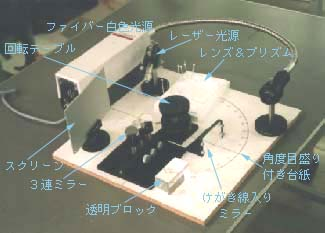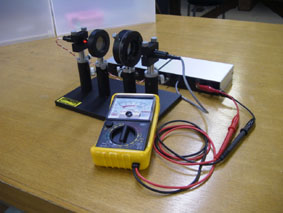# Education

1. Fresnel reflectance experiment set

By using two types of prisms with high and low refractive indices as test samples, the difference in Fresnel reflectance of materials with different refractive indices can be easily measured and tested. In addition, since the test geometry is a prism, the refractive index of the sample can be experimentally determined by using the prism method (minimum declination method, etc.). Furthermore, by adding another polarizer unit ( optional ), the instrument can be used as a simple ( introductory ) polarization-related experimental system.

2. Introduction to Optics Experiment Kit: OPTKITThis kit was developed by Uniopt based on the idea of Professor Kawabata of the Physics Laboratory at Tokyo Polytechnic University. It has been adopted as a theme for physics experiments. With this kit, you can intuitively experience the reflection and refraction of light. A variety of experiments can be performed by combining the kit. The kit has been adopted not only by Tokyo Polytechnic University but also by Osaka Sangyo University. Click here for more details.

3. Diffraction Experiment Set : DEFKIT
In this experiment, students will observe the diffraction of light. Students will learn the basics of how light interacts with objects and how the state of objects can be predicted and calculated using diffraction phenomena. In the experiments, students can learn the following. This course has been adopted by the Faculty of Engineering, Yamagata University.

• To experience diffraction phenomena and to understand the basic knowledge of diffraction by using diffraction gratings around us.
• When an object (slit or circular aperture) that changes the amplitude of light spatially is placed in the optical path, the changes in the light produced by passing through the object are observed, and the width of the slit and the diameter of the circular aperture are determined from the measurement of the Fraunhofer diffraction pattern.
• To observe the changes in the light passing through an object that changes its phase spatially (phase grating), and to consider the structure of the object from some diffraction patterns.

4. Polarization Experiment Set: POLKITPolarization and birefringence of light can be experienced intuitively with this experiment set. A wide variety of experiments can be performed by combining different devices. By polarizing the light emitted by the LED, the experiment becomes more fun and you can experience the change in polarization state (wavelength dispersion of birefringence) due to the difference in color. In addition, students can learn how different colors change the polarization state (wavelength dispersion of birefringence). This system has been adopted by the Tokyo Polytechnic University, Faculty of Engineering, and Osaka Sangyo University.

5. Lens prism experimental kit: LNSKIT
This experimental kit is a set of translucent lenses and prisms. The translucent optical components allow us to see the optical path of light passing through them. Each optic has a magnet embedded in it, and by using a special magnetic base plate, the kit can be used for various applications such as demonstrations with the plate in an upright position. The standard shapes of the optical components are convex lens, concave lens, right-angle prism, equilateral triangle prism, and parallelogram prism, but various shapes are available upon request.